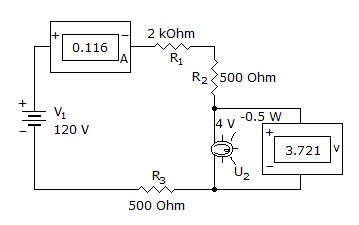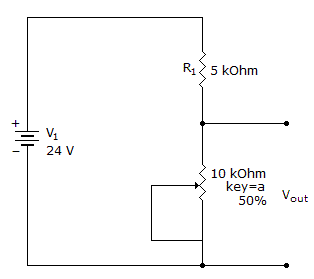# Electronics - Series Circuits

### Exercise :: Series Circuits - General Questions

11.

An 8-ohm resistor is in series with a lamp. The circuit current is 1 A. With 20 V applied, what voltage is being allowed for the lamp?

 A. 4 V B. 8 V C. 12 V D. 20 V

Explanation:

No answer description available for this question. Let us discuss.

12.

What is wrong, if anything, with this circuit?A. R1 is open. B. R1 is shorted. C. R2 is open. D. R2 is shorted.

Explanation:

No answer description available for this question. Let us discuss.

13.

Kirchhoff’s voltage law states that:

 A. the sum of the voltage drops in a series circuit is equal to the total applied voltage B. the algebraic sum of the resistances is equal to the sum of the voltages C. the algebraic sum of the individual currents around a closed loop is zero D. the voltages developed across each element in a series circuit are identical

Explanation:

No answer description available for this question. Let us discuss.

14.

If series current doubles, then:

 A. resistance is halved B. voltage is doubled C. voltage is reduced D. resistance is doubled

Explanation:

No answer description available for this question. Let us discuss.

15.

What are the minimum and maximum output voltages?A. 0 V, 4 V B. 0 V, 8 V C. 0 V, 12 V D. 0 V, 16 V